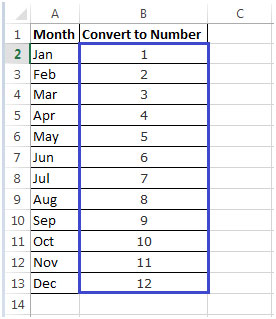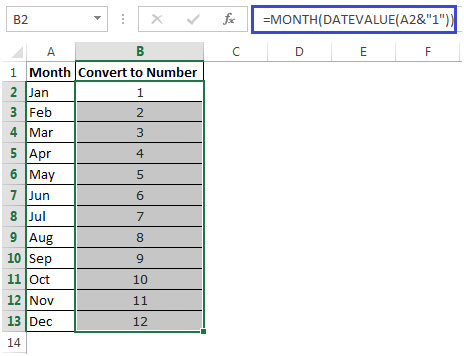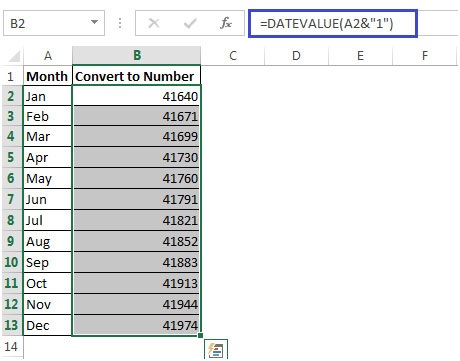Converting Month Name to a Number in Microsoft Excel

In this article we will learn how to convert month name to a number in Microsoft Excel 2010.

While preparing reports there could be a requirement to convert a column that holds the name of the month (January, February, etc) into a number (1, 2).

Let us understand with an Example:

• Column A contains Months Jan, Feb, Mar till Dec.
• We want to convert the Months to a Number. i.e. Jan=1, Feb =2 & so on as shown below which has been input manually.We can use combination of MONTH & DATEVALUE functions to get result.

• We can use the following formula in cell B2& then copy down to B3:B13 as shown in the below picture to get the output
• In cell B2=MONTH(DATEVALUE(A2&”1″))• The DateValue formula will convert the Month say Jan to 41640. If we format the number 41640 to Date Format, it will return 1/1/2014• Enclosing the Datevalue formula in Month Formula will convert the number 41640 to 1.
• Thus, we get the required outputIn this way we can convert name of month to the number of month.

## Users are saying about us...

1.Or =1*(1&A2) and format cell as “mmm”
or =Month(1*(1&A2)

Terms and Conditions of use

The applications/code on this site are distributed as is and without warranties or liability. In no event shall the owner of the copyrights, or the authors of the applications/code be liable for any loss of profit, any problems or any damage resulting from the use or evaluation of the applications/code.